Mobile QR Code## I. INTRODUCTION

Recently, the demand for power-efficient, wide dynamic range (> 100 dB) microphone read-out IC (M-ROIC) is increasing in mobile or automotive industries. M-ROIC consists of a programmable gain amplifier (PGA) and an ADC as shown in Fig. 1 (1-4). To achieve an M-ROIC of above 100 dB dynamic range (DR), both the PGA and the ADC should have more than 100 dB DR.

For the ADC, delta-sigma (DS) ADC has been widely employed in M-ROIC because of its power-efficient, high DR characteristic (5). Especially, continuous-time (CT) DS ADCs are attractive to high precision mobile audio applications because of their inherent anti-aliasing characteristics and power efficiency compared to their discrete-time DS counterparts (6-8). However, a low-power wide DR CT DS ADC is difficult to implement because of the non-linearities arising from the circuit building blocks such as inter-symbol interference of the DAC, non-linearity of the OTA and etc. Several researches have reported high linearity CT DS ADCs employing dedicated circuit techniques to overcome such difficulties like balancing the rising/falling time of the DAC (6), negative-resistors at the OTA input (7) or zoom structures (8). But such methods usually lead to additional power consumption, area occupation or increased design complexity.

Usually, a wide DR also implies a high SNR. However, in some application, a very high SNR might not be necessary, whereas a very wide DR is required. In those applications, a wide DR M-ROIC can be achieved with an ADC of moderate performance by automatic gain control (AGC) of the PGA (3,4). Then, the area and the power consumption for the ADC can be greatly saved.

In this brief, we present a design of an AGC circuit for the M-ROIC. The AGC was designed to maintain large amplitude at the ADC input while minimizing the nonlinear distortion from the saturation of the PGA in the event of a sudden increase of the input to M-ROIC. The AGC used a high-frequency-clock-operated gain-down circuit and a low-frequency-clock-operated gain-up circuit to achieve low power consumption and minimal signal clipping simultaneously.

Fig. 2. Dynamic range of the AGC M-ROIC (Solid line: 5-level variable gain pre-amplifier, dotted line: fixed gain pre-amplifier).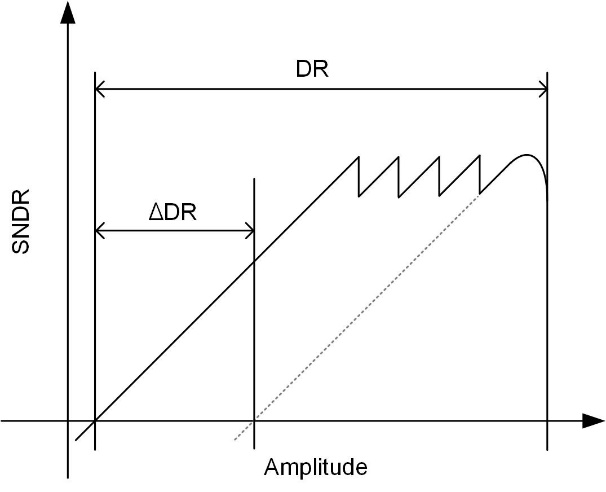AGCs are also commonly used in radio-frequency wireless communications systems where the input signal amplitude can vary widely (9-11). In those systems, however, the high-frequency nature of the carrier favors the use of different kinds of signal strength measurement methods.

To verify the operation of the proposed AGC system, we performed SPICE-level simulations employing a PGA and a CT DS ADC and obtained 101.2 dB DR from the AGC M-ROIC with the ADC of 82.5 dB SNR, which represents a DR extension of 18 dB. This confirms that we can obtain wide DR employing an ADC of moderate SNR.

This brief is organized as follows. In section II, the operation principles and DR extension of AGC M-ROIC is described. In section III, we present the design of a AGC, and in section IV, we demonstrate the feasibility of the AGC technique for the M-ROIC by SPICE-level simulations. Finally, we conclude in section V.

## II. Architecture

Fig. 2 shows the principle of the DR extension by using PGA gain control. The solid line shows SNR versus signal amplitude of the AGC M-ROIC where the PGA gain has 5 levels, whereas the dashed line represents the SNR when a fixed pre-amplifier gain was used. In Fig. 2, with the PGA gain steps of 6 dB, we have a DR extension of 24 dB. In general, when the number of gain levels is $\textit{N}$ and a gain step is $\textit{A}$ dB, we can obtain a DR extension of ($\textit{N}$-1)x$\textit{A}$ dB.

Fig. 3. Structures of AGC M-ROIC which detect the signal amplitude (a) at the input of the PGA (Type 1), (b) at the output of the ADC (Type 2), (c) at the output of the PGA (Type 3).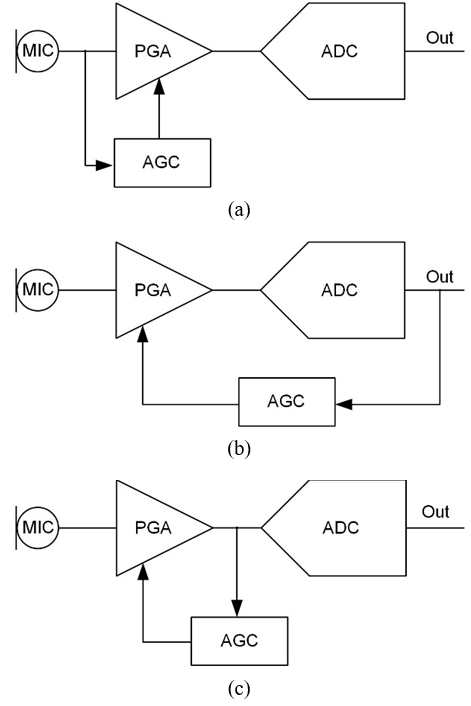The above full DR extension is possible only if the noise of the ADC dominates over that of the PGA. In practice, as the PGA gain is increased, the output noise power of the PGA is increased, which can reduce the DR extension. In general, the SNR of the AGC M-ROIC is

##### (1)
$SNR=10\log _{10}\frac{G^{2}P_{sig,in}}{G^{2}P_{ni,PGA}+P_{n,ADC}}$,

where $P_{sig,in}$ is the input signal power, $\textit{P}$$_{ni\_PGA} is the PGA input referred noise power, P_{n,ADC} is the ADC output noise power and \textit{G} is the gain of the PGA. Here, we assumed that the signal transfer function (STF) of the ADC is unity. In (1) , we can observe that if G^{2}P_{ni,PGA} = P_{n,ADC}, then by increasing \textit{G} by a factor of 2 we can increase the SNR by 6 dB. However, if G^{2}P_{ni,PGA} ? P_{n,ADC}, increasing \textit{G} does not improve SNR. Therefore, the proposed DR extension scheme is applicable only when the realization of a low noise PGA is less challenging than that of a low noise ADC. Fig. 4. Waveforms of AGC M-ROIC in Fig. 3 (a) input to the PGA, (b) output of PGA (Type 2), (c) output of PGA (Type 3).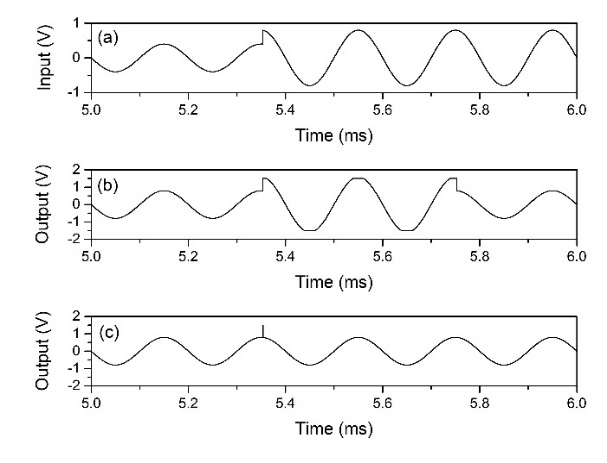In an M-ROIC using an AGC, the AGC block senses the signal amplitude and adjusts the gain of the PGA accordingly. Fig. 3 shows three possible structures of the M-ROIC employing an AGC. In Fig. 3(a), the AGC measures the signal amplitude at the input of the PGA (Type 1), and in Fig. 3(b) the AGC measures the amplitude at the output of the ADC (Type 2). In Fig. 3(c), the signal amplitude is measured at the output of PGA (Type 3). An advantage of the Type 2 AGC is that the sensing mechanism is all digital and the sensing circuit does not load the analog output of the PGA. However, it suffers from extra delay between the input and output of the ADC. Usually, DS ADCs are employed in high precision audio applications. When a DS ADC is used, the DS modulator output should be filtered by a decimation filter before the signal amplitude can be measured. Therefore, the delay of an ADC can be significant. When the signal amplitude is being reduced, the delay will lead to temporary SNR degradation. However, when the input of the M-ROIC increases suddenly and saturates the PGA output, the delay will cause large nonlinear distortion of the PGA output. Fig. 4 shows the waveform of the input and output of the PGA. Fig. 4(a) shows the input of the PGA and Fig. 4(b) shows the output of the PGA when Type 2 AGC is used. Fig. 4(b) illustrates the saturation of the PGA output after the input signal is suddenly increased by a large factor before the new gain level is applied. Because the Type 3 AGC senses the analog output of PGA directly, it does not suffer from the large ADC delay. Fig. 4(c) shows the output of PGA for Type 3 AGC. We can observe a very short pulse when the input amplitude suddenly increases. The duration of the pulse is determined by the delays of the AGC and PGA and can be as short as micro-seconds. This short pulse can be easily filtered-out by the decimation filter of ADC. A disadvantage of Type 3 AGC is that it loads the PGA output. However, because the required resolution of the sensing circuit in the AGC is very low compared to the resolution of the ADC, the loading can be tolerated. Fig. 5. Waveform of the three signals with different amplitude. The reference voltages representing the upper or the lower limit are also shown.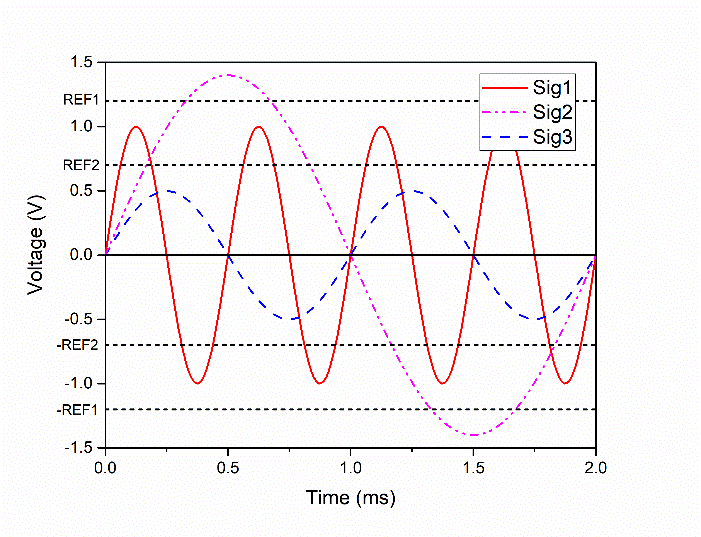Type 1 AGC senses the PGA input directly. An advantage is that the delay between the signal amplitude change and the PGA gain change is minimized because the signal is sensed even before it enters the PGA. However, in this scheme, to determine the signal amplitude range in which the signal amplitude is, we need multi-bit ADC as the sensing circuit. When the gain-levels follow geometric sequence as in our case, the ADC accuracy should be very high when the input signal level is very small. This will burden our system design. Considering these pros and cons, we decided to use Type 3 architecture in our work. ## III. AGC System Design Now, we present the design of the AGC logic circuit and sensing circuit. Fig. 5 shows three signals of different amplitude along with reference voltages used in the AGC to determine the signal amplitude. The \textit{V}$$_{\mathrm{Ref1}}$ represents the upper limit of the signal and $\textit{V}$$_{\mathrm{Ref2}} represents the lower limit. In Fig. 5, the peaks of Sig1 are between \textit{V}$$_{\mathrm{Ref1}}$ and $\textit{V}$$_{\mathrm{Ref2}} (or between the {-}$$\textit{V}$$_{\mathrm{Ref1}} and {-}$$\textit{V}$$_{\mathrm{Ref2}}), and we should not change the PGA gain. The peaks of Sig2 are larger than \textit{V}$$_{\mathrm{Ref1}}$ (or smaller than ${-}$$\textit{V}$$_{\mathrm{Ref1}}$). It means that the signal is too large and the PGA gain should be lowered. Finally, Sig3 stays between the $\textit{V}$$_{\mathrm{Ref2}} and {-}$$\textit{V}$$_{\mathrm{Ref2}}, and the PGA gain should be increased. Fig. 6. Flow-chart representing the PGA gain setting algorithm.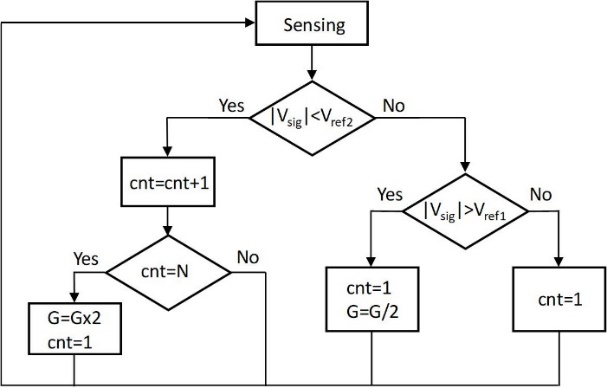\textit{V}$$_{\mathrm{Ref2}}$ should be a little smaller than a half of $\textit{V}$$_{\mathrm{Ref1}} to avoid repeated up and down switchings of the PGA gain. To maximize SNR, \textit{V}$$_{\mathrm{Ref1}}$ should be maximized. However, if it is too large, the PGA output will suffer from nonlinear distortion. Furthermore, the no-overload condition of the DS ADC also limits the useful range of $\textit{V}$$_{\mathrm{Ref1}}. In this work, \textit{V}$$_{\mathrm{Ref1}}$ = 0.86 $\textit{V}$$_{\mathrm{DD}}, and \textit{V}$$_{\mathrm{Ref2}}$ = 0.42 $\textit{V}$$_{\mathrm{DD}}, where \textit{V}$$_{\mathrm{DD}}$ = 1.5 V.

It is easy to determine whether the PGA gain should be lowered or not by comparing the instantaneous value of the PGA output signal with $\textit{V}$$_{\mathrm{Ref1 }}(and {-}$$\textit{V}$$_{\mathrm{Ref1}}). However, when we determine whether the PGA gain should be increased, it is not enough to compare the instantaneous value of the signal with \textit{V}$$_{\mathrm{Ref2 }}$(and ${-}$$\textit{V}$$_{\mathrm{Ref2}}$), because even large signals pass through the region between $\textit{V}$$_{\mathrm{Ref2}} and {-}$$\textit{V}$$_{\mathrm{Ref2}} as illustrated by all three signal waveforms in Fig. 5. To distinguish a small signal from a large signal temporarily passing through the low voltage region, we employed a counter. If the sensing circuit determines that the instantaneous value of the signal is small, the counter output is increased by one, but the gain is not changed yet. Only after we have \textit{N} consecutive “Small” flags from the sensing circuit, the PGA gain is increased to the next level and the counter is reset. And whenever the “Small” flag is OFF, the counter is reset. Fig. 6 shows the algorithm used in this work to set the PGA gain. Fig. 7. Simplified schematic diagram of (a) the AGC circuit, (b) the clock generation circuit.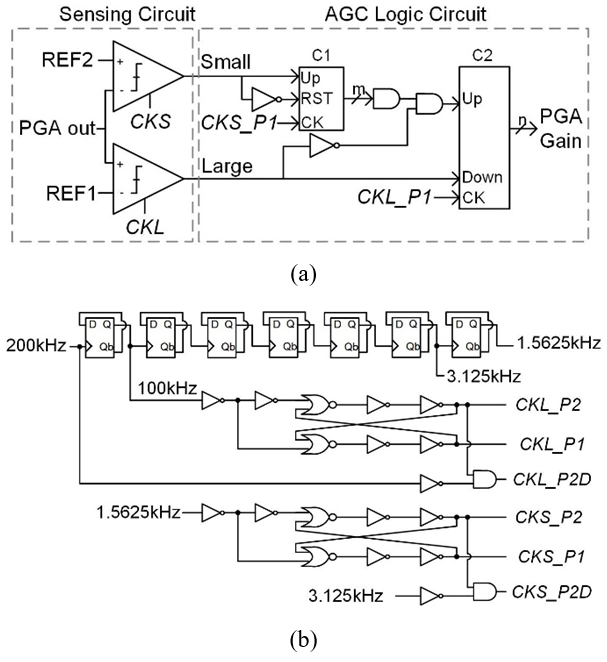The number of consecutive “Small” flags required to increase the PGA gain, \textit{N}$$_{small}$, should be determined considering the signal frequency and the delay resulting from the counter operation. $\textit{N}$$_{small} should be large enough so that large-amplitude-low-frequency signals should be able to pass from \textit{V}$$_{\mathrm{Ref2}}$ to ${-}$$\textit{V}$$_{\mathrm{Ref2}}$ in less than $\textit{N}$$_{small} comparisons. From this we obtain ##### (2) N_{\textit{small}}>\frac{f_{\textit{sense}}}{2f_{sig,min}}, where f_{\textit{sense}} and 2f_{sig,min} represent the AGC clock frequency and the minimum signal frequency. On the other hand, if \textit{N}$$_{small}$ is too large, it leads to unnecessarily long delays before the PGA gain is increased. During the delay, the signal supplied to the ADC is small, and the M-ROIC suffers from SNR reduction. Considering the trade-off between the SNR degradation and the sensing accuracy, we used $\textit{f}$$_{\mathrm{sense}} = 1.5625 kHz and N_{\textit{small}}=2^{5}, which corresponds to the time delay of 20.5 ms and f_{sig,min}=24.4Hz\,. It should be noted that during this delay the signal does not suffer devastating clipping as it does during the delay associated with the gain decrease after sudden increase of signal amplitude. It just suffers from temporary SNR degradation. Fig. 7(a) shows the schematic diagram of the AGC circuit. For simplicity, only two reference voltages \textit{V}$$_{\mathrm{Ref1}}$ and $\textit{V}$$_{\mathrm{Ref2}} are shown and their negative counterparts are omitted. (The actual sensing circuits are shown in Fig. 8). Fig. 7(b) shows the clock generation circuit for the AGC circuit which consists of a clock divider and two non-overlap clock generators. The \textit{CKS} (or \textit{CKL}) in the Fig. 7(a) represents \textit{CKS_P1}, \textit{CKS_P2} and \textit{CKS_P2D} collectively (or \textit{CKL_P1}, \textit{CKL_P2} and \textit{CKL_P2D}) in Fig. 7(b). Two clocked comparators in the sensing circuit compare the instantaneous value of the input signal with \textit{V}$$_{\mathrm{Ref1}}$ and $\textit{V}$$_{\mathrm{Ref2}}. If the sensing circuit produces a “Large” flag, the PGA gain is reduced to the next lower level immediately at the next rising edge of \textit{CKL_P1}. The time interval between the occurrence of the “Large” flag and the next rising edge of \textit{CKL_P1} causes the PGA output to saturate as illustrated in Fig. 4(c) which can lead to very large signal clipping. To minimize the clipping, we used relatively high frequency clocks of 100 kHz for \textit{CKL}. Fig. 8. Block diagrams of the proposed sensing circuits for (a) the “Large” flag, (b) the “Small” flag.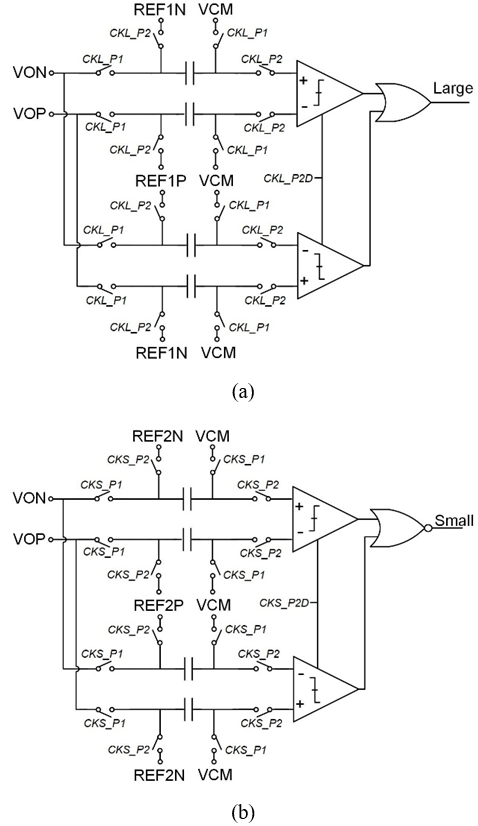If the sensing circuit produces a “Small” flag, then the \textit{m}-bit counter C_{1} is up-counted by one. If the counter output reaches \textit{N} = 2^{\mathrm{m}}, then the PGA gain is increased to the next higher level. For \textit{CKS}, we used 1.5625 kHz as mentioned previously. Table 1. Target specifications  Parameters Target specifications VDD 1.5 V Bandwidth 20 kHz Peak PGA SNR 100 dB Peak ADC SNR 82 dB PGA gains 1, 2, 4, 8 DR 100 dB Expected DR extension 18 dB For the detectors, differential-comparison is suitable to sense the signal level accurately which could avoid the sensing error caused by the offset of the PGA or common mode noises. For the differential comparison, 4-input comparators are widely adopted (12). However, when the reference voltage is very large as in our case, it is difficult to design a 4-input comparator. Therefore, we designed a switched-capacitor (SC) sensing circuits using 2-input comparators, which are shown in Fig. 8. The V_{\mathrm{OP}} and the V_{\mathrm{ON}} represent the positive and negative outputs of the PGA. The circuit in Fig. 8(a) determines if the signal (V_{\mathrm{sig}}=V_{\mathrm{OP}}-V_{\mathrm{ON}}) is too large (|V_{\mathrm{sig}}| > V_{\mathrm{Ref1}} (=V_{\mathrm{Ref1P }}$${-}$ V$_{\mathrm{Ref1N}}$)) and the one in Fig. 8(b) determines if the signal is too small (|V$_{\mathrm{sig}}$| < V$_{\mathrm{Ref2}}$ (=V$_{\mathrm{Ref2P }}$${-} V_{\mathrm{Ref2N}})). As 2-input clocked comparators in Fig. 8, strong-arm comparators were used (3). ## IV. Simulation We demonstrate the operation of the AGC M-ROIC by Spectre simulations of a ROIC system consisting of a PGA, CT-DS ADC and AGC using a 28 nm CMOS process. The target peak-SNR and the DR of the M-ROIC was 80 dB and 100 dB, respectively, in the 20 kHz bandwidth (BW). The PGA has gain from 1 (0 dB) to 8 (18 dB) to obtain an 18 dB DR extension. Target design specifications are summarized in Table 1. Fig. 9 shows the structure of the PGA used in this brief. The PGA adopted a resistive negative-feedback structure where the PGA gains were determined by the ratio of the input resistor and feedback resistors. The gain is programmable from 0 dB to 18 dB with steps of 6 dB by selecting a feedback resistor from a bank of resistors. There are two kinds of noise sources in the PGA, which are the resistors and the OTA. We allocate half of the total PGA noise to the resistors and the rest to the OTA when the PGA gain was 1 (0 dB). For this, we choose the input resistance of 10 kΩ. Fig. 9. Structural diagram of PGA with AGC circuits and ADC.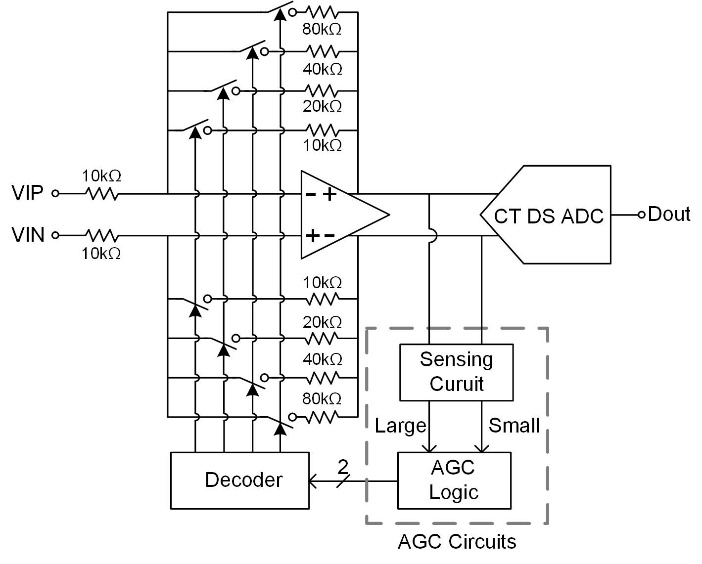Fig. 10 shows the schematic diagram of the 2-stage Miller-compensated OTA for the PGA. To suppress the flicker noise, we used large transistors for the first stage. The DC gain of the OTA was 78 dB and the unity-gain frequency was 14.5 MHz while consuming 300 μA current. As discussed in Sec. II, the noise of PGA is important for effective DR extension. Fig. 11 shows the input referred noise of the OTA and the PGA when the PGA gain was 1 (0 dB) from ac noise simulations. From the results, we could obtain the integrated input referred noise of PGA in the 20 kHz BW of 2.5x10^{-11} V^{2}, which is much smaller than that of the 80 dB DR ADC with 1 V full scale of about 1x10^{-9} V^{2}. Also we performed transient noise simulations of the PGA and obtained 104.2 dB SNR and 100.8 SNDR. These results confirm that the PGA performance is sufficient for the 100 dB DR M-ROIC. Fig. 11. Simulation results of the input referred noise of the OTA and the PGA.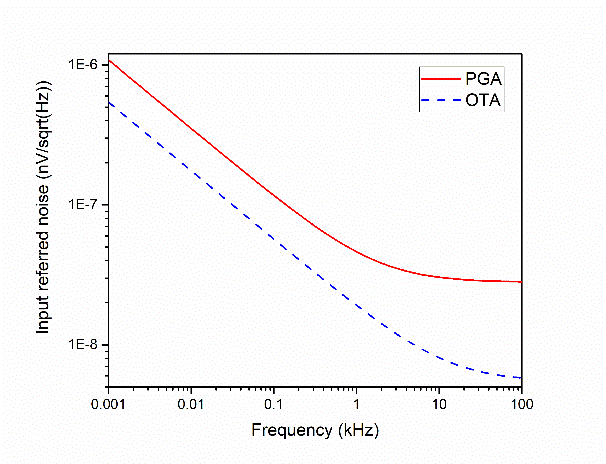Fig. 12. Simplified single-ended schematic diagram of the ADC.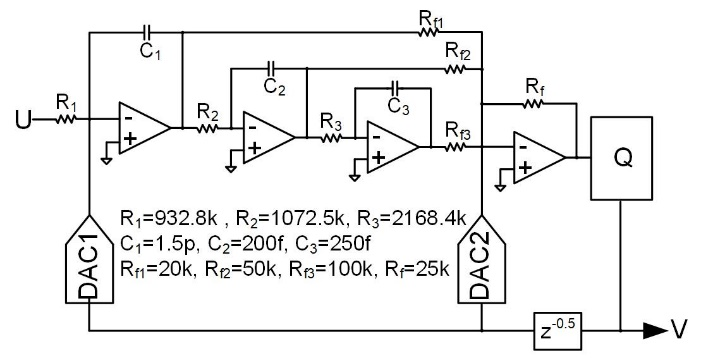Fig. 12 shows the schematic diagram of the ADC used in this brief along with the value of components. We employed a CT-DS ADC with a 3rd-order feedforward loop filter and a 1-bit quantizer. The OSR of the ADC was 128 and the sampling frequency was 6.4 MHz. The NTF of the ADC is ##### (3)$$ N T F(z)=\frac{(z-1)^{3}}{(z-0.6694)\left(z^{2}-1.531 z+0.6639\right)}$$To compensate the excess loop delay, DAC2 was employed. In this work, the CT DS ADC was implemented using ideal OTAs and an ideal quantizer focusing on the behavior of the AGC circuits and PGA. The maximum SQNR obtained from Spectre simulations excluding circuit noise were 98.8 dB. In the simulations verifying the DR extension, we included the noise of resistors. With resistor noise included, the maximum SNR was reduced to 83.7 dB. Fig. 13 shows the Spectre simulation results. Fig. 13(a) shows the input of the PGA and Fig. 13(b) shows the output. In Fig. 13(b), the red dashed lines represent V$_{\mathrm{Ref1}}$and${-}$V$_{\mathrm{Ref1}}$, and the blue dash-dot-dot lines represent V$_{\mathrm{Ref2}}$and${-}$V$_{\mathrm{Ref2}}$. Initially the PGA gain was 0 dB and the input of the PGA was small. In this condition, the output amplitude of the PGA is smaller than V$_{\mathrm{Ref2}}$and the AGC logic increases the PGA gain every 20.5 ms. After 61.5 ms, the output amplitude of the PGA stays between V$_{\mathrm{Ref2}}$and V$_{\mathrm{Ref1}}$. Fig. 13(c) shows the corresponding increase of the PGA gain. At T = 150 ms, the input amplitude of the M-ROIC is suddenly increased from 30 mV to 500 mV and the amplitude of the PGA output becomes larger than V$_{\mathrm{Ref2}}$almost instantaneously. Then, the sensing circuit senses the output signal level change and lowers the PGA gain successively until the output amplitude becomes smaller than V$_{\mathrm{Ref2}}$. Unlike the slow increase of the gain from 0 dB to 18 dB, the reduction of the gain from 18 dB to 6 dB is very fast. The waveform of the output of the PGA at around T = 150 ms is enlarged in Fig. 13(d). We can observe two successive gain changes clearly. Fig. 13. Simulation results of PGA with proposed AGC Logic. (a) PGA input waveform, (b) PGA output waveform, (c) PGA gain profile, (d) a zoomed-in of PGA output waveform of (b) around T=150 ms.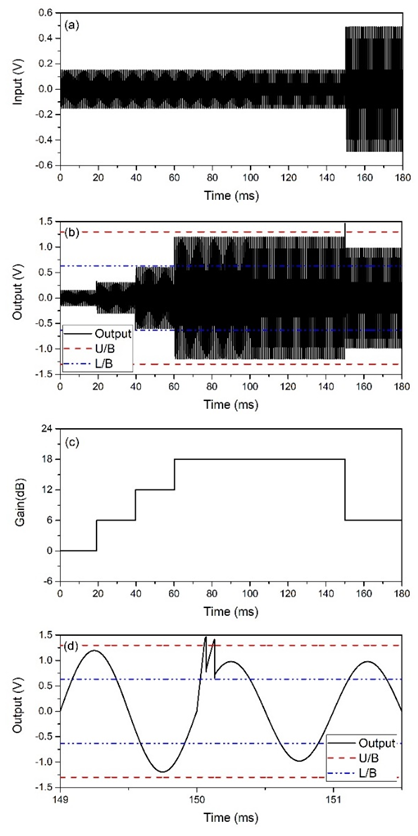Fig. 14. SNDR of the AGC M-ROIC versus input amplitude obtained by simulations.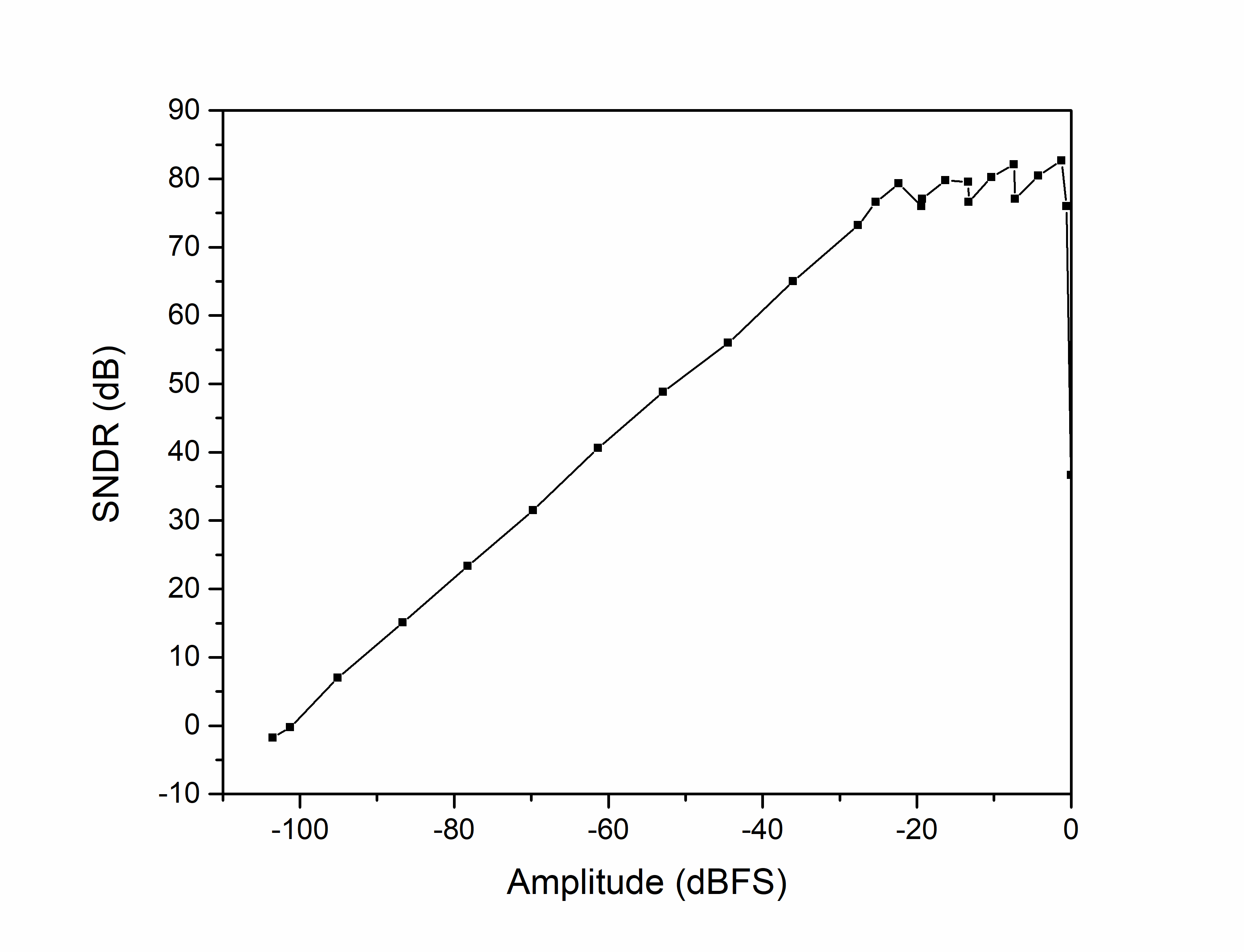Table 2. Comparison between the AGC Logic circuits  This work (1) (13) Architecture AGC PGA + ADC PGA + ADC + DAC Pre-amp Process 28 nm 0.13 μm 65 nm Bandwidth 20 kHz 10 kHz 20 kHz Power Consumption 450 μW+ 290 μW 185 μW Peak SNR 82.6 dB 79 dB 88.6 dB DR 101.2 dB 106 dB ---$^{+}\$: Power consumption of ADC not included.

Fig. 14 shows the SNDR versus input amplitude of the proposed AGC M-ROIC with various the input signal amplitude. The PGA gain is determined by the AGC circuits according to the input signal level. From the simulations, we obtained 101.2 dB DR with the ADC whose SNR was 83.7 dB, which represents 18 dB of DR extension.

Comparisons of the M-ROIC performance are provided in Table 2. Note that our work focused on the AGC, and a DS ADC with ideal OTAs and an ideal comparator was used in simulations. Therefore, the power consumption of the ADC was not included. Considering the bandwidth, peak SNR, and DR, our work shows competitive power efficiency among the comparison.

## V. Conclusions

In this brief, we presented a wide DR M-ROIC employing an AGC. The proposed AGC circuits consist of a PGA-output-amplitude sensing circuit and an AGC logic circuit. For the sensing circuit, we proposed an SC sensing circuit which accurately sensed the signal level and produced the signals for the AGC circuit. Then the AGC determined the PGA gain by manipulating the signals from the sensing circuit. The proposed AGC circuit was verified by Spectre simulations of an M-ROIC employing a PGA and a DS ADC. The simulation results confirmed that the DR of the M-ROIC was successfully extended.

### ACKNOWLEDGMENTS

This research was supported by the KIAT (Korea Institute for Advancement of Technology) grant funded by the Korea Government (MOTIE). (No. N0001883, The competency development program for industry specialist) The CAD tools were provided by IC Design Center (IDEC), Korea.

### REFERENCES

1
Sukumaran A., Karanjkar K., Jhanwar S., Krishnapura N., Pavan S., Nov 2012, A 1.2 V 285 μA analog front end chip for a digital hearing aid in 0.13 μm CMOS, in Proc. of IEEE Asian Solid-State Circuits Conf. (A-SSCC), pp. 397-4002
Im S., Park S.-G., Nov 2016, A 154-μW 80-dB SNDR Analog-to-digital front-end for digital hearing aids, Wiley Analog integrated Circuits and Signal Processing, Vol. 89, No. 2, pp. 383-3935
Pavan S., Schreier R., Temes G. C., 2017, Understanding delta-sigma data converters, 2nd Ed., IEEE Press – Wiley, Hoboken, NJ, USA6
Theertham R., Koottala P., Billa S., Pavan S., July 2019, A 24 mW Chopped CTDSM Achieving 103.5 dB SNDR and 107.5 dB DR in a 250 kHz bandwidth, in Proc. of IEEE Symposium on VLSI circuits, pp. C226-C2277
Jang M., Lee C., Chae Y., Jan 2019, Analysis and design of low-power continuous-time delta-sigma modulator using negative-R assisted Integrator, IEEE J. of Solid-State Circuits, Vol. 54, No. 1, pp. 277-2878
Gonen B., Karmakar S., Veldhoven R., Makinwa K. A. A., Apr 2020, A Continuous-time zoom ADC for low-power audio applications, IEEE J. of Solid-State Circuits, Vol. 55, No. 4, pp. 1023-10319
Jimenez V. P. G., Garcia M. J. F.-G., Serrano F. J. G., Armada A. G., Nov 2004, Design and implementation of synchronization and AGC for OFDM-based WLAN receivers, IEEE Trans. Consumer Electronics, Vol. 50, No. 4, pp. 1016-102510
Wang X., Chi B., Wang Z., Aug 2010, A low-power high-data-rate ASK IF receiver with a digital-control AGC loop, IEEE Trans. Circuits and Systems II, Vol. 57, No. 8, pp. 617-62111
Pérez J. P. A., Calvo B., Celma S., Aug 2010, A high-performance CMOS feedforward AGC circuit for a WLAN receiver, IEEE Trans. Industrial Electron., Vol. 57, No. 8, pp. 2851-285712
Cho T. B., Gray P. R., Mar 1995, A 10 b, 20 Msample.s, 35 mW Pipeline A/D Converter, IEEE J. Solid-State Circuits, Vol. 30, No. 3, pp. 166-17213
Song S., Lee C., Jang M., Chae Y., 2019, A 185 μW −105.1 dB THD 88.6 dB SNDR negative-R stabilized audio preamplifier, in Proc. European Solid-state circuits Conf. (ESSCIRC)## Author

Saemin Im received the B.S. and M.S. degrees in Electronics Engi-neering from Hanyang University, Seoul, Korea, in 2010 and 2013, respectively and is currently working towards the Ph.D. degree in the same university. His research area is mixed-signal CMOS integrated circuits and memory interface circuits.

Na-Hoo Lee received the B.S. degree in Electronics Engineering from Gangneung-Wonjo National University, Gangneung, Korea in 2018 and received the M.S. degree in Electronics Engineering from Hanyang University, Seoul, Korea in 2020. His research area is the mixed-signal CMOS circuit design, with focus on delta-sigma oversampling data converters.

Kyoungho Baik was born in Seoul, Korea. He received B.S degree in the School of Electronics Engineering from Soongsil University, Korea, in 2019. He is currently pursuing the M.S. degree in the Electronics Engi-neering from Hanyang University, Korea. His interests include Delta-sigma ADCs.

Sang-Gyu Park received B.S. and M.S. degrees in Electronics Engi-neering from Seoul National Univer-sity in 1990 and 1992, respectively and received Ph.D. degree in Electrical and Computer Engineering from Purdue University in 1998. He worked at AT&T Laboratories-Research from 1998 to 2000 and joined the faculty of Hanyang University in 2000, where he is a professor in Electronics and Computer Engineering. His research area is the mixed-signal CMOS circuit design, with focus on delta-sigma oversampling data converters, high speed SAR ADCs and memory interface circuits.